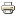# Distance rationalizability of scoring rulesWorking paper
Author/s:
Burak Can
Issue number:
68/2013
Series:
GSBE
Publisher:
Maastricht University
Year:
2013
Collective decision making problems can be seen as finding an outcome that is closest to a concept of consensus. 1 introduced Closeness to Unanimity Procedure as a first example to this approach and showed that the Borda rule is the closest to unanimity under swap distance a.k.a the 2 distance. 3 shows that the Dodgson rule is the closest to Condorcet under swap distance. 4, 5 generalized this concept as distance-rationalizability, where being close is measured via various distance functions and with many concepts of consensus, e.g., unanimity, Condorcet etc. In this paper, we show that all non-degenerate scoring rules can be distance-rationalized as Closeness to Unanimity procedures under a class of weighted distance functions introduced in 6. Therefore, the results herein generalizes 1 and builds a connection between scoring rules and a generalization of the Kemeny distance, i.e. weighted distances.
Developed by Paolo Gittoi